Mathematical and Physical Journal
for High Schools
Issued by the MATFUND Foundation
 Already signed up? New to KöMaL?

# KöMaL Problems in Physics, March 2007

Show/hide problems of signs:## Problems with sign 'M'

Deadline expired on April 11, 2007.

M. 277. Measure in which case will a cup of milk coffee be colder: if cold milk is poured into the hot coffee immediately and then the mixture is left to cool down for a while, or if the hot coffee is left to cool for the same time as in the previous case, and then cold milk is is added.

(6 pont)

statistics## Problems with sign 'P'

Deadline expired on April 11, 2007.

P. 3963. Find the mass of the object which is to be raised to a height of 1 metre such that the change in its energy is the same as the absorbed energy when 1 litre of water is heated by.

(3 pont)

statistics

P. 3964. Where does the linear momentum of the swinging child come from? (Initially she is sitting on the swing without moving and her feet do not touch the ground.)

(4 pont)

statistics

P. 3965. From the top of a rough sphere of radius R a small ball of radius r and of mass m rolls down with no initial speed. Where will the ball begin to slide and what is the frictional force at the moment when it begins to slide if R=1 m, r=0.1 m, m=15 kg, and the coefficient of static friction is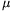=0=0.2?

(5 pont)

statistics

P. 3966. Two waves of the same amplitude are generated by two point-like wave sources. The waves are in phase, their frequency is 5 Hz and their speed is 2 m/s. What can you observe at the point which is 40 cm from one of the sources and 60 cm from the other.

(4 pont)

statistics

P. 3967. In a container there is mixture of helium and hydrogen gas. What is the ratio of the masses of the two gases if the partial pressures of the two gases are equal.

(4 pont)

statistics

P. 3968. The tap in the garden was closed at 8 o'clock in the evening, but unfortunately it was closed inappropriately. The water flowing out of it forms a downward narrowing beam as shown in the figure. The beam of water has a circular cross-section, the diameter of the circle is 6 mm at one point and 4 cm below it is only 3.4 mm. How much water was wasted if the tap is closed properly at 8 o'clock next morning. (The viscosity of the flowing water can be neglected.)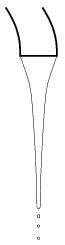(5 pont)

statistics

P. 3969. Electric currents of magnitude I flow in two loops made of insulated wires, their direction is shown in the figure. The shapes of the loops are regular n-gons. In which case will the magnetic field be greater at the centre of circle that is circumscribed around the n-gon?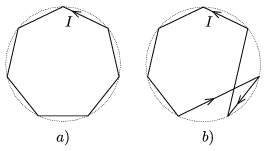(5 pont)

statistics

P. 3970. An electron is shot into uniform magnetic field of magnitude B=10-3 T the width of which is d=2 cm. The velocity of the electron is perpendicular to the magnetic field lines, the angle between the velocity of the electron and the normal of the boundary is=30o.

a) What is the least velocity of the electron if it goes through the region of magnetic field of width d?

b) What is the speed below which the electron cannot go through the magnetic field of width d?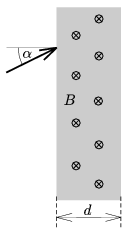(5 pont)

statistics

P. 3971. In 1611, before the law of refraction was discovered by Snell, Kepler published the book Dioptrice in which he describes his investigation about the refraction of light entering from air into a rock-crystal. He found that the angle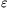between the extension of the incident ray and the refracted ray is proportional to the angle of incidence:, where k is a constant (in case of the rock crystal).

a) Verify Kepler's formula for small angles.

b) Aim a beam of monochromatic light to a rock crystal prism the apex angle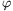of which is small, such that the beam is nearly perpendicular to the surface of the prism. Using Kepler's formula determine the angle between the incident ray and the ray which leaves the prism.

(5 pont)

statistics

P. 3972. With what percentage will the shortest wavelength of a continuous x-ray spectrum change if the voltage of the x-ray tube is increased by 10%?

(4 pont)

statistics